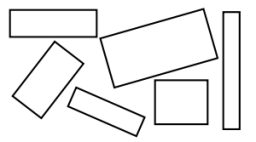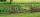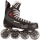# Percent change

If the length of a rectangle is increased by 25% and the width is decreased by 10%, the area of the rectangle is larger than the area of the original rectangle by what percent?

p =  12.5 %

### Step-by-step explanation:Did you find an error or inaccuracy? Feel free to write us. Thank you!Tips to related online calculators

#### You need to know the following knowledge to solve this word math problem:

We encourage you to watch this tutorial video on this math problem:

## Related math problems and questions:

• Hop-gardenThe length of the rectangular hop garden Mr. Smith increased by 25% and its width by 30%. What is the percentage change in area of hop garden?
• Rectangle areaThe length of a rectangle of x units is increased by 10%, and its width of y units is increased by 15%. What is the ratio of the area of the old rectangle to the area of the new rectangle?
• PlotThe length of the rectangle is 8, smaller than three times the width. If we increase the width by 5% of the length and the length is reduced by 14% of the width, the circumference of the rectangle will be increased by 30 m. What are the dimensions of the
• Profit growthThe profit of a company increased by 25% during the year 1992, increased by 40% during the year 1993, decreased by 20% in 1994 and increased by 10% during the year 1995. Find the average growth in the profit level over the four years periods?
• The hallThe hall had a rectangular ground plan one dimension 20 m longer than the other. After rebuilding the length of the hall declined by 5 m and the width has increased by 10 m. Floor area increased by 300 m2. What were the original dimensions of the hall?
• RectangleFind the dimensions of the rectangle, whose perimeter is 108 cm and the length is 25% larger than the width.
• Area of gardenIf the width of the rectangular garden is decreased by 2 meters and its length is increased by 5 meters, the area of the rectangle will be 0.2 ares larger. If the width and the length of the garden will increase by 3 meters, its original size will increas
• ParcelBoth dimensions of the rectangular parcel were increased by 31%. By how many % has increased its acreage?
• PropertyThe length of the rectangle-shaped property is 8 meters less than three times of the width. If we increase the width 5% of a length and lendth reduce by 14% of the width it will increase the property perimeter by 13 meters. How much will the property cost
• SquareSuppose the square's sides' length decreases by a 25% decrease in the content area of 28 cm2. Determine the side length of the original square.
• Ice skatesIce skates were raised twice, the first time by 25%, the second time by 10%. After the second price, their cost was 82.5 euros. What was the original price of skates?
• Unknown number 10Number first increased by 30%, then by 1/5. What percentage we've increased the original number?
• Percentage and rectangleAbout what percentage increases perimeter and area of a rectangle if both the sides 12 cm and 10 cm long we increase by 20%?
• Percents from percentHow much is 13% of 20% of 500 greater than 8% of 14% of 200?
• Price increase 2xIf two consecutive times we increase the price of the product by 20%, how many % is higher final price than the original?
• Content area and percentsDetermine what percentage is smaller cube surface, when the surface area of the wall decreases by 25%.
• Washing machineThe washing machine cost € 325. In sale-off, they became cheaper by 10%, later by another 10%. Find the last price of the washing machine.